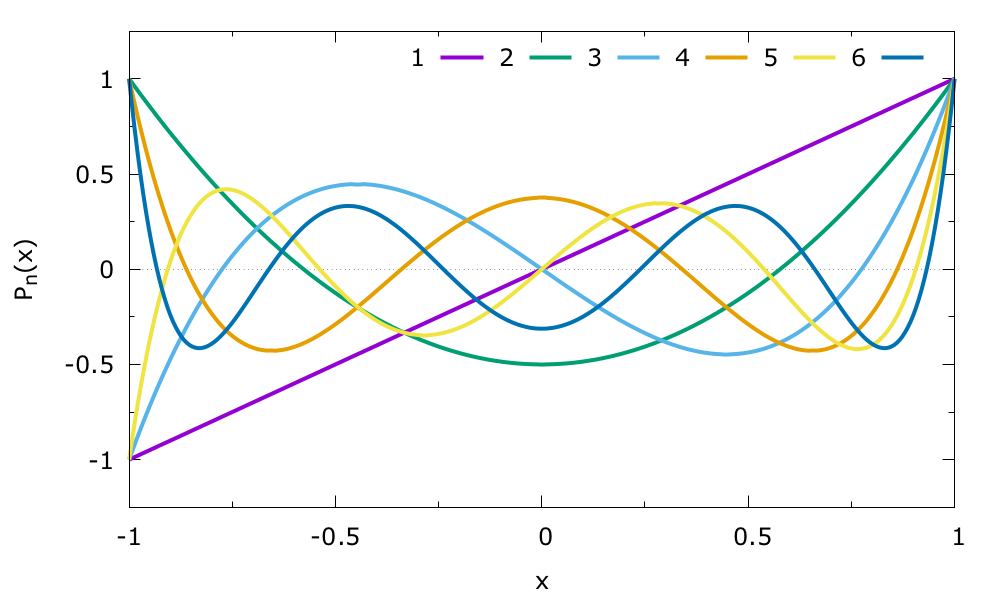BF_LEGENDRE

Legendre polynomials basis functions.

Use as basis functions Legendre polynomials $$P_{n}(x)$$ defined on a bounded interval. You need to provide the interval $$[a,b]$$ on which the basis functions are to be used, and the order of the expansion $$N$$ (i.e. the highest order polynomial used). The total number of basis functions is $$N+1$$ as the constant $$P_{0}(x)=1$$ is also included. These basis functions should not be used for periodic CVs.

Intrinsically the Legendre polynomials are defined on the interval $$[-1,1]$$. A variable $$t$$ in the interval $$[a,b]$$ is transformed to a variable $$x$$ in the intrinsic interval $$[-1,1]$$ by using the transform function

$x(t) = \frac{t-(a+b)/2} {(b-a)/2}$

The Legendre polynomials are given by the recurrence relation

\begin{align} P_{0}(x) &= 1 \\ P_{1}(x) &= x \\ P_{n+1}(x) &= \frac{2n+1}{n+1} \, x \, P_{n}(x) - \frac{n}{n+1} \, P_{n-1}(x) \end{align}

The first 6 polynomials are shown belowThe Legendre polynomial are orthogonal over the interval $$[-1,1]$$

$\int_{-1}^{1} dx \, P_{n}(x)\, P_{m}(x) = \frac{2}{2n+1} \delta_{n,m}$

By using the SCALED keyword the polynomials are scaled by a factor of $$\sqrt{\frac{2n+1}{2}}$$ such that they are orthonormal to 1.

From the above equation it follows that integral of the basis functions over the uniform target distribution $$p_{\mathrm{u}}(x)$$ are given by

$\int_{-1}^{1} dx \, P_{n}(x) p_{\mathrm{u}}(x) = \delta_{n,0},$

and thus always zero except for the constant $$P_{0}(x)=1$$.

For further mathematical properties of the Legendre polynomials see for example the Wikipedia page.

Examples

Here we employ a Legendre expansion of order 20 over the interval -4.0 to 8.0. This results in a total number of 21 basis functions. The label used to identify the basis function action can then be referenced later on in the input file.

Click on the labels of the actions for more information on what each action computesbf_leg: BF_LEGENDRE MINIMUMcompulsory keyword
The minimum of the interval on which the basis functions are defined. =-4.0 MAXIMUMcompulsory keyword
The maximum of the interval on which the basis functions are defined. =8.0 ORDERcompulsory keyword
The order of the basis function expansion. =20

Examples
Glossary of keywords and components
Compulsory keywords
 ORDER The order of the basis function expansion. MINIMUM The minimum of the interval on which the basis functions are defined. MAXIMUM The maximum of the interval on which the basis functions are defined.
Options
 DEBUG_INFO ( default=off ) Print out more detailed information about the basis set. Useful for debugging. NUMERICAL_INTEGRALS ( default=off ) Calculate basis function integral for the uniform distribution numerically. Useful for debugging. SCALED ( default=off ) Scale the polynomials such that they are orthonormal to 1.